# Induced connection on submanifold

## Definition

### For a submanifold of a Riemannian manifold

Suppose$M$ is a Riemannian manifold,$N$ is a submanifold. Then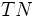$TN$ is a subbundle of the bundle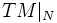$TM|_N$. The Riemannian metric on$M$ naturally gives a notion of "projection" from$TM|_N$ to$TN$.

Then, given any linear connection$\nabla$ on$M$, we obtain an induced connection$\nabla|_N$ on$N$. The induced connection is defined as follows. For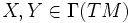$X,Y \in \Gamma(TM)$, we have: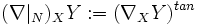$(\nabla|_N)_X Y := (\nabla_XY)^{tan}$

The definition has two key parts:

1. We use a connection on$TM$ to obtain a connection on$TM|_N$. This can be done either by using the general notion of a pullback connection, or by the following more concrete process: First, using the tubular neighborhood theorem, consider a tubular neighborhood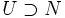$U \supset N$ in$M$. Extend$X,Y$ to vector fields on$U$ by Fill this in later. Evaluate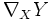$\nabla_XY$ on this open set$U$, to get a vector field on$U$. Restrict the vector field to$N$. This gives a section of the bundle$TM|_N$
2. We project this from$TM|_N$ to$TN$, using the Riemannian metric

Crudely speaking, we use the connection on$M$, and project the output vector field we get, onto$TN$.